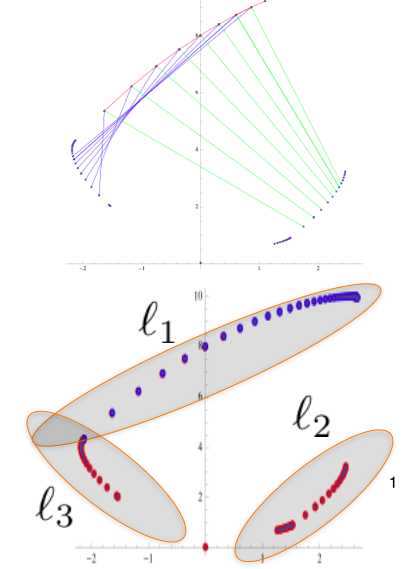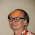Tuesday, July 29, 2014

Fractals: parameters estimation (part IV)

Introduction
In the former posts we discussed about the following points:
1. There are special points in the contour of the fractal that can be used to derive its contour.
2. Such points can be used to describe the fractal thru an iterative and deterministic algorithm.
It's still open the main issue: can we leverage the two above findings to determine the fractals parameters?
The answer is yes, provided that we use a good technique to extract the contour points of the fractal image.

The algorithm (practical explanation)
We already noticed that points in the contour of the fractal are in tight relationship each other.
Since each linear transformation is described by exactly six parameters, for their determination we need at least 6 points for each transformation function.
The below image makes the concept clear:Image-1: Relationship between points of the fractal contour

The steps to obtain the estimation of the params are the following:
1. Extraction of the contour points thru convex hull algorithm;
2. Build relationships among the points.Left Image: Extraction of contour points thru Convex Hull. Right Image: Relationship among the points extracted.
How to build the relationships among the points?
As showed both in the deterministic algorithm presented in the last post and in the above image, the points preserve an ordering that can be leveraged to simplify the complexity of the problem.
Consider for instance the fern case:
• the transformation that leads to the fixed point lying on the contour (highlighted in image-1) can be obtained (as explained in the points 1,2 of the deterministic algorithm) just creating the relationship among the consecutive points of the set $l_1$ described in Image-1 and in the below image:Set of points to determine the Transformations
At this point we obtained the parameters of the first transformation, but still, we don't know neither the number of transformations we really need to describe the fractal image,  nor the probability associated to them.
For the first problem, we have to strategies: the brute force, that is, try all the possible combinations, or more wisely try the soft computing approach described below.

The fuzzy clustering approach (...unfortunately here some formulas are required)
The fuzzy clustering is a very well known algorithm that assigns the membership of a point to a cluster with a certain "degree", so the point $p_i$ might belong to the cluster $A$ with degree $x$ and to the cluster $B$ with degree $y$.

In this problem I modified the algorithm in this way:
• the clusters are the mapping functions that we want to discover.
• the probability associated to the fractal maps can be easily derived by the "size" of each cluster.
• The fuzzy-membership function is based on the minimisation of the distance between the point obtained applying to $x_i$ the contractive map $x_i^{'} = \tau_j(x_i)$  and all the other points falling in a reasonable neighbour. The animation below describes it.

• The update step of the centroids is aimed to minimise the distance between $d(\tau (x_i)),\phi(x_i,\tau_j))$ computed over each $\tau$ and each $x_i$, where
• $\phi(x_i,\tau_j) = \arg\min_{x_s} d(\tau_j(x_i),x_s)$
• To minimise the above function,  I used the gradient technique, working on the the following cost function:
• $E(\theta)= \sum_{i=1}^{n}{(\mu_{x_i}(\tau_j)\cdot[d(\tau_j(x_i),\phi(x_i,\tau_j))]^2)}$
• For each mapping function,  the correction to each param is the following:
• $\theta_i= \theta_i - \eta \frac{\partial E(\theta)}{\partial \theta_i}$

Results
Starting from the image of the fern (a jpg image containing 100.000 points) the application of the algorithm for the determination of the contractive maps gives the following result:Contractive maps detection algorithm: in black the points of the original fern, in red,green, blue the three estimated maps.
• The results are not bad, the weakness of the algorithm lays on the extraction of the points of the Convex hull of the fractal.
• The application of smarter contour extraction algorithm should improve further the accuracy of the algorithm.
Disclaimer
In the last posts about fractals I often abused the term contour of fractal. ...Theoretically speaking, a fractal cannot have a contour, but to make clear the pragmatical aspects I decided voluntarily such terminology.

1.2.3.